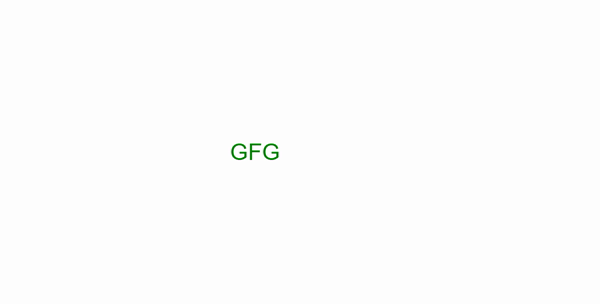# Explosion Animation in Canvas

• Last Updated : 01 Feb, 2021

Explosion animation can be created using canvas in HTML.

Approach: At first, we have to create a canvas element in HTML and get its reference in JavaScript. All the elements and animations are controlled using JavaScript functions. Some steps required are:

Hey geek! The constant emerging technologies in the world of web development always keeps the excitement for this subject through the roof. But before you tackle the big projects, we suggest you start by learning the basics. Kickstart your web development journey by learning JS concepts with our JavaScript Course. Now at it's lowest price ever!

• Use the canvas methods to get the canvas and the context.
• Decide the color, pattern, and gradient for text and particle object.
• Decide and draw the shape for animation or frame rendering
• Clear the previous canvas.
• Save the canvas after transformations for the original state
• Restore the canvas before drawing new frame
We can create many particles and animate them in random directions which fades out after some time.

HTML code:

## HTML

 ```<``html``>`` ` `<``head``>``    ``<``style``>``        ``* {``            ``padding: 0;``            ``margin: 0;``            ``box-sizing: border-box;``            ``;``        ``}``         ` `        ``body {``            ``overflow: hidden;``        ``}``    `````` ` `<``body``>`` ` `    ``<``canvas` `id``=``"myCanvas"``>`` ` `    ``<``script``>`` ` `        ``/* Get the canvas  */``        ``var canvas = document.getElementById("myCanvas");``         ` `        ``/* Get the height and width of the window */``        ``canvas.height = window.innerHeight;``        ``canvas.width = window.innerWidth;``         ` `        ``/* Get the 2D context of the canvas  */``        ``var ctx = canvas.getContext("2d");``         ` `        ``/* Fills or sets the color,gradient,pattern */``        ``ctx.fillStyle = "white";``        ``ctx.fillRect(0, 0, canvas.width, canvas.height);``        ``ctx.font = "50px Arial";``        ``ctx.fillStyle = "green";``         ` `        ``/* Writes the required text  */``        ``ctx.fillText("GFG", 500, 350)``        ``let particles = [];``         ` `        ``/* Initialize particle object  */``        ``class Particle {``            ``constructor(x, y, radius, dx, dy) {``                ``this.x = x;``                ``this.y = y;``                ``this.radius = radius;``                ``this.dx = dx;``                ``this.dy = dy;``                ``this.alpha = 1;``            ``}``            ``draw() {``                ``ctx.save();``                ``ctx.globalAlpha = this.alpha;``                ``ctx.fillStyle = 'green';``                 ` `                ``/* Begins or reset the path for ``                   ``the arc created */``                ``ctx.beginPath();``                 ` `                ``/* Some curve is created*/``                ``ctx.arc(this.x, this.y, this.radius, ``                        ``0, Math.PI * 2, false);`` ` `                ``ctx.fill();``                 ` `                ``/* Restore the recent canvas context*/``                ``ctx.restore();``            ``}``            ``update() {``                ``this.draw();``                ``this.alpha -= 0.01;``                ``this.x += this.dx;``                ``this.y += this.dy;``            ``}``        ``}``         ` `        ``/* Timer is set for particle push ``            ``execution in intervals*/``        ``setTimeout(() => {``            ``for (i = 0; i <= 150; i++) {``                ``let dx = (Math.random() - 0.5) * (Math.random() * 6);``                ``let dy = (Math.random() - 0.5) * (Math.random() * 6);``                ``let radius = Math.random() * 3;``                ``let particle = new Particle(575, 375, radius, dx, dy);``                 ` `                ``/* Adds new items like particle*/``                ``particles.push(particle);``            ``}``            ``explode();``        ``}, 3000);`` ` `        ``/* Particle explosion function */``        ``function explode() {`` ` `            ``/* Clears the given pixels in the rectangle */``            ``ctx.clearRect(0, 0, canvas.width, canvas.height);``            ``ctx.fillStyle = "white";``            ``ctx.fillRect(0, 0, canvas.width, canvas.height);``            ``particles.forEach((particle, i) => {``                    ``if (particle.alpha <= 0) {``                        ``particles.splice(i, 1);``                    ``} else particle.update()``                ``})``                 ` `                ``/* Performs a animation after request*/``            ``requestAnimationFrame(explode);``        ``}``    `````` ` `        `

Output:Explosion Animation

My Personal Notes arrow_drop_up